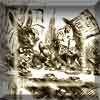You may also likeA Problem of Time

Consider a watch face which has identical hands and identical marks for the hours. It is opposite to a mirror. When is the time as read direct and in the mirror exactly the same between 6 and 7?Tricircle

The centre of the larger circle is at the midpoint of one side of an equilateral triangle and the circle touches the other two sides of the triangle. A smaller circle touches the larger circle and two sides of the triangle. If the small circle has radius 1 unit find the radius of the larger circle.Two Triangles in a Square

Given that ABCD is a square, M is the mid point of AD and CP is perpendicular to MB with P on MB, prove DP = DC.

Surprising Transformations

Why do this problem?

This problem helps students to consolidate their understanding of how equations of the form $y=mx+c$ describe the gradient and position of lines. Students explore the effect of combining reflections and translations and justify why a pair of transformations is (or isn't) commutative.

This is good preparation for future work on transforming the graphs of more complicated functions.

Possible approach

This printable worksheet may be useful: Surprising Transformations

This builds on Translating Lines and Reflecting Lines.

Start by introducing the problem. Hand out this set of transformation cards and suggest that students work in pairs, recording the order of transformations they have tried, and the eventual outcomes. Encourage students to work in rough, sketching just the essential properties of the lines at each stage. Allow some working time before bringing the class together.

Suggest to the class that findings could be shared, and organise the board so that all the results can be displayed: eventual outcomes and the corresponding transformations. Invite students to record the results they have so far. In order to avoid mistakes creeping in, suggest students tick any results they can confirm.

Allow some more working time, with students adding to the record of results. The class may wish to organise themselves so that different groups are allocated the job of investigating particular combinations.

Questions that may emerge:
How do we know that we have considered all the possible combinations?
Why are all the eventual outcomes parallel to each other?
How many different outcomes are possible?
Could we have predicted the number of different outcomes in advance?
Why do certain combinations produce the same outcome as others?

This can lead to a discussion about commutativity: why does the order matter for some pairs of transformations but not for others? Once students have established which pairs of transformations are commutative, it is worth returning to the results on the board to appreciate why some combinations led to the same eventual outcome.

Key questions

How does each transformation affect the gradient and the intercept?
Why do some pairs of transformations produce the same outcome regardless of the order?

Possible extension

Students could consider what happens when the same four transformations are applied to different starting graphs. What similarities are there between results? Can they be explained?

Students could also consider the effect on a graph of rotation through multiples of 90 degrees and investigate what happens when these are combined with translations and reflections.

Possible support

Ensure that students are secure about the relationship between a line's properties and its equation. Encourage students to sketch the graphs of different equations and then use the interactivity below to check their work.

Full Screen Version